Updating search results...

# 38 Results

View
Selected filters:
• Look At Physics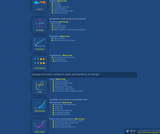Conditional Remix & Share Permitted
CC BY-SA
Rating
0.0 stars

In this video, we explore some concepts fundamental to algebra. To streamline the discussion of relationships between physical quantities, we introduce variables, functions, composition, and inverse. By thinking about the concept of an inverse function, we obtain our first glimpse of the imaginary root (i.e. square-root of -1) and the complex plane.

Subject:
Physics
Material Type:
Lecture Notes
Provider:
Look At Physics
Provider Set:
A Mathematical Way to Think About Biology
Author:
David Liao
10/08/2011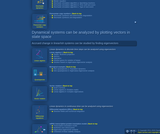Conditional Remix & Share Permitted
CC BY-SA
Rating
0.0 stars

In the master-equation formalism, a set of differential equations describe the time-evolution of the probability distribution of an ensemble of systems. This can be used, for example, to describe the varied mRNA copy numbers found in individual cells in a population.

Subject:
Physics
Material Type:
Lecture Notes
Provider:
Look At Physics
Provider Set:
A Mathematical Way to Think About Biology
Author:
David Liao
10/08/2012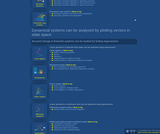Conditional Remix & Share Permitted
CC BY-SA
Rating
0.0 stars

The stochastic simulation algorithm (SSA, Kinetic Monte Carlo, Gillespie algorithm) produces an example trajectory for a particular member of a probabilistic ensemble by looping over the following steps. The current state of the system is used to determine the likelihood of each possible chemical reaction in relative comparison to the likelihoods for the other possible reactions, as well as to determine when the next reaction is expected. Pseudo-random numbers are drawn to "roll the dice" to determine exactly when the next reaction will proceed, and which kind of reaction it will happen to be.

Subject:
Physics
Material Type:
Lecture Notes
Provider:
Look At Physics
Provider Set:
A Mathematical Way to Think About Biology
Author:
David Liao
10/08/2012Conditional Remix & Share Permitted
CC BY-SA
Rating
0.0 stars

In the first part of this video introduction to combinatorics, we introduce factorial notation to efficiently describe the ways we can arrange distinguishable objects in a row. Next, we count the combinations of L objects that can be grabbed from a container of L + N total objects. Finally, we use the formula for combinations to derive the binomial theorem.

Subject:
Physics
Material Type:
Lecture Notes
Provider:
Look At Physics
Provider Set:
A Mathematical Way to Think About Biology
Author:
David Liao
10/08/2012Conditional Remix & Share Permitted
CC BY-SA
Rating
0.0 stars

In this deterministic cellular automata model, interactions between neighboring automata are described using a prisoner's dilemma. Limiting dispersal of seeds of annual plants can permit heterogeneous co-existence, whereas thorough mixing instead allows one subpopulation to dominate quickly.

Subject:
Physics
Material Type:
Lecture Notes
Provider:
Look At Physics
Provider Set:
A Mathematical Way to Think About Biology
Author:
David Liao
10/08/2012Conditional Remix & Share Permitted
CC BY-SA
Rating
0.0 stars

In this video, we use direction fields (drawn as quiver plots) to illustrate the numerical integration of differential equations. We include a heuristic example of how one might try to adapt step size by comparing different orders of approximation.

Subject:
Physics
Material Type:
Lecture Notes
Provider:
Look At Physics
Provider Set:
A Mathematical Way to Think About Biology
Author:
David Liao
10/08/2012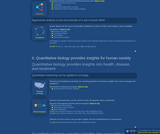Conditional Remix & Share Permitted
CC BY-SA
Rating
0.0 stars

In the first video, we present a biological circuit topology from Ma, Trusina, El-Samad, Lim, and Tang, "Defining network topologies that can achieve biochemical adaptation," Cell, 138: 760-773 (2009). This topology supports adaptation, which is not the absence of change in response to stimulation/stress, but, instead, the ability to produce delayed compensation for those changes. In the second video, we summarize the method of almost linear stability analysis used to solve for the dynamics of this example system.

Subject:
Physics
Material Type:
Lecture Notes
Provider:
Look At Physics
Provider Set:
A Mathematical Way to Think About Biology
Author:
David Liao
10/08/2012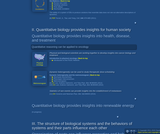Conditional Remix & Share Permitted
CC BY-SA
Rating
0.0 stars

To describe how oscillations are supported in systems of differential equations, we present a classic "Romeo and Juliet" picture of two-dimensional oscillations, and we analyze how trajectories change as nullclines are arranged at different angles in the phase plane. In addition to models based on traditional systems of differential equations, dynamical systems with time delays and dynamical systems with stochastic fluctuation (i.e. stochastic resonance) can also support oscillations.

Subject:
Physics
Material Type:
Lecture Notes
Provider:
Look At Physics
Provider Set:
A Mathematical Way to Think About Biology
Author:
David Liao
10/08/2012Conditional Remix & Share Permitted
CC BY-SA
Rating
0.0 stars

In the five parts of this video, we define the derivative and then build a cribsheet of rules for expressing the slopes of simple functions and combinations of functions. These include the power rule, the chain rule, the product and quotient rules, and the rules for differentiating sinusoidal functions.

Subject:
Physics
Material Type:
Lecture Notes
Provider:
Look At Physics
Provider Set:
A Mathematical Way to Think About Biology
Author:
David Liao
10/08/2012Conditional Remix & Share Permitted
CC BY-SA
Rating
0.0 stars

The first video segment presents a canonical mathematical example from quantitative biology, in which mRNA is transcribed from a gene sequence, and protein is translated from mRNA. The second segment uses eigenvector-eigenvalue analysis to sketch the trajectories of the system in a phase portrait. Finally, the third segment generalizes the linear stability analysis used to study this example.

Subject:
Physics
Material Type:
Lecture Notes
Provider:
Look At Physics
Provider Set:
A Mathematical Way to Think About Biology
Author:
David Liao
10/08/2012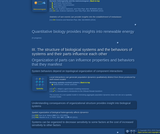Conditional Remix & Share Permitted
CC BY-SA
Rating
0.0 stars

Biological systems (e.g. cells) can make stochastic transitions between phenotypes (e.g. states of relatively increased or decreased drug resistance). This means that an initially drug-sensitive population can generate relatively drug-resistant subpopulations. This video presents a metronomogram, which is a tool for understanding whether such stochastic transitions can provide an opportunity for therapeutic treatment. Citation: Liao D, Estevez-Salmeron L, and Tlsty TD (2012) "Conceptualizing a tool to optimize therapy based on dynamic heterogeneity," Phys. Biol. 9:065005.

Subject:
Physics
Material Type:
Lecture Notes
Provider:
Look At Physics
Provider Set:
A Mathematical Way to Think About Biology
Author:
David Liao
10/08/2012Conditional Remix & Share Permitted
CC BY-SA
Rating
0.0 stars

We express the exponential function of an imaginary variable in terms of sine and cosine. The "complex exponentials" that result trace out a circle in the complex plane. Pointing to one of the positions in the complex plane, we obtain the identity exp(i pi) = -1.

Subject:
Physics
Material Type:
Lecture Notes
Provider:
Look At Physics
Provider Set:
A Mathematical Way to Think About Biology
Author:
David Liao
10/08/2012Conditional Remix & Share Permitted
CC BY-SA
Rating
0.0 stars

In the first video segment, we introduce Euler's number by considering the problem of interest compounded continuously. After we obtain the power-series representation for exp(x), we explore its properties, in the next four video segments, to convince ourselves that exp(x) is literally an exponential function, meaning a number, approximately 2.71828, taken to the power x. In the final two segments, we present the natural logarithm and demonstrate that it is the anti-derivative of 1/x.

Subject:
Physics
Material Type:
Lecture Notes
Provider:
Look At Physics
Provider Set:
A Mathematical Way to Think About Biology
Author:
David Liao
10/08/2011Conditional Remix & Share Permitted
CC BY-SA
Rating
0.0 stars

In the first video segment, we analyze the population dynamics for a test-tube of cells that affect each others' likelihoods of replication when they collide. The particular example we use is a prisoner's dilemma, which has the almost paradoxical property that survival of the relatively most fit leads overall fitness to decrease. In the second video segment, we suggest that the population dynamics from the first segment can be related to an analysis that uses payoff matrices found in traditional game theory.

Subject:
Physics
Material Type:
Lecture Notes
Provider:
Look At Physics
Provider Set:
A Mathematical Way to Think About Biology
Author:
David Liao
10/08/2012Conditional Remix & Share Permitted
CC BY-SA
Rating
0.0 stars

In the first part of this video, we review the idea of flat space and the Pythagorean theorem. The second part of the video reviews ideas from trigonometry including the unit circle, sine, cosine, and pi. We conclude with a proof of an angle-sum identity.

Subject:
Physics
Material Type:
Lecture Notes
Provider:
Look At Physics
Provider Set:
A Mathematical Way to Think About Biology
Author:
David Liao
10/08/2011Conditional Remix & Share Permitted
CC BY-SA
Rating
0.0 stars

In the first video segment, we present a cartoon model of a weighted chain, which can be regarded as an approximation for a polymer under tension (e.g. a strand of DNA being stretched out using optical tweezers). The Hamiltonian and partition function for this system are described in the second segment. Finally, in the third segment, we calculate the average energy and elongation of the chain.

Subject:
Physics
Material Type:
Lecture Notes
Provider:
Look At Physics
Provider Set:
A Mathematical Way to Think About Biology
Author:
David Liao
10/08/2012Conditional Remix & Share Permitted
CC BY-SA
Rating
0.0 stars

In this video, we become familiar with integrals, both by understanding them as sums of areas under plots of functions, and by understanding them as anti-derivatives. We present the u-substitution rule, which is the analog of the chain rule for differentiating composite functions.

Subject:
Physics
Material Type:
Lecture Notes
Provider:
Look At Physics
Provider Set:
A Mathematical Way to Think About Biology
Author:
David Liao
10/08/2011Conditional Remix & Share Permitted
CC BY-SA
Rating
0.0 stars

Students will see how the idea of a limit can be presented both in formal epsilon-delta-style terms, and using corresponding animations. After calculating a limit for a simple example function, we point out that limits do not always exist.

Subject:
Physics
Material Type:
Lecture Notes
Provider:
Look At Physics
Provider Set:
A Mathematical Way to Think About Biology
Author:
David Liao
10/08/2012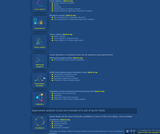Conditional Remix & Share Permitted
CC BY-SA
Rating
0.0 stars

The first video segment presents a "freemium" business model as a motivating example. The second and third segments provide mathematical background on vectors, vector spaces, operators, and representations. Using this theoretical foundation, we solve for the dynamics of the example business using eigenvalue-eigenvector analysis.

Subject:
Physics
Material Type:
Lecture Notes
Provider:
Look At Physics
Provider Set:
A Mathematical Way to Think About Biology
Author:
David Liao
10/08/2012Conditional Remix & Share Permitted
CC BY-SA
Rating
0.0 stars

Using the complex exponentials described in the previous video, we show how oscillating systems can be modeled using "rotation matrices." One strategy for determining whether a dynamical system supports oscillations is to look for complex eigenvectors and eigenvalues.

Subject:
Physics
Material Type:
Lecture Notes
Provider:
Look At Physics
Provider Set:
A Mathematical Way to Think About Biology
Author:
David Liao
10/08/2012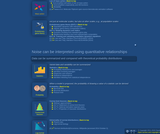Conditional Remix & Share Permitted
CC BY-SA
Rating
0.0 stars

In the first part of this video, we derive the law of mass action from one example of a picture of molecular collisions. For this course, we use the "law of mass action" to refer to an idea that chemical reaction kinetic rates can be expressed using products of the abundances of reactants raised to exponents. Studying cooperativity and Hill functions in the second part of the video allows us to investigate a simple example of bistability in the third video segment.

Subject:
Physics
Material Type:
Lecture Notes
Provider:
Look At Physics
Provider Set:
A Mathematical Way to Think About Biology
Author:
David Liao
10/08/2012Conditional Remix & Share Permitted
CC BY-SA
Rating
0.0 stars

The purpose of this video tutorial is to review a couple ways in which we think about numbers. Thinking in terms of street numbers, money in bank accounts, and quantum particles (e.g. Bose-Einstein condensate) is contrasted with focusing on associating numbers with distinguishable manipulatives, as is more familiar in K-8 courses. This video concludes with a reminder that the symbol "infinity" is not, itself, a number.

Subject:
Physics
Material Type:
Lecture Notes
Provider:
Look At Physics
Provider Set:
A Mathematical Way to Think About Biology
Author:
David Liao
10/08/2011Conditional Remix & Share Permitted
CC BY-SA
Rating
0.0 stars

When a function depends on multiple independent variables, the curly-d symbol denotes slopes calculated by jiggling only one independent variable at a time. This is a multivariable cousin to the derivative. We use this notation in future sections to keep track of how molecules are generated or degraded by different reactions.

Subject:
Physics
Material Type:
Lecture Notes
Provider:
Look At Physics
Provider Set:
A Mathematical Way to Think About Biology
Author:
David Liao
10/08/2012Conditional Remix & Share Permitted
CC BY-SA
Rating
0.0 stars

Why do quantitative biologists sometimes claim that mRNA copy numbers are Poisson distributed in simple models of gene transcription? The first video segment addresses this question under the simplifying assumption that mRNA degradation occurs after a well-defined, deterministic lifetime, and the second segment illustrates the same basic concept for the more realistic situation in which degradation is stochastic.

Subject:
Physics
Material Type:
Lecture Notes
Provider:
Look At Physics
Provider Set:
A Mathematical Way to Think About Biology
Author:
David Liao
10/08/2013Conditional Remix & Share Permitted
CC BY-SA
Rating
0.0 stars

After heuristically deriving Stirling's approximation in the first video segment, we outline a simple example of the central limit theorem for the case of the binomial distribution. In the final segment, we explain how the central limit theorem is used to suggest that physical experiments are characterized by normally-distributed (Gaussian) fluctuations while fluctuations in biological experiments are said to fill out log-normal distributions.

Subject:
Physics
Material Type:
Lecture Notes
Provider:
Look At Physics
Provider Set:
A Mathematical Way to Think About Biology
Author:
David Liao
10/08/2012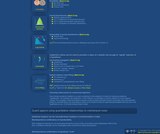Conditional Remix & Share Permitted
CC BY-SA
Rating
0.0 stars

In the first video segment, we study the distribution, average, and variance for the Bernoulli coin-toss process. The binomial distribution results from stringing together a series of coin tosses. In the second segment, we study the limit of "rare" events, which is described by the Poisson distribution.

Subject:
Physics
Material Type:
Lecture Notes
Provider:
Look At Physics
Provider Set:
A Mathematical Way to Think About Biology
Author:
David Liao
10/08/2012Conditional Remix & Share Permitted
CC BY-SA
Rating
0.0 stars

The quadratic formula is easy to solve, yet sufficiently sophisticated that it provides insight into oscillations of masses connected by springs, as well as insight into chemical bonds between atoms. The purpose of this video is to illustrate what it means to find the "zeros" or "roots" of the quadratic equation, both using a graphical description, as well as by analytically completing the square to obtain the famous quadratic formula.

Subject:
Physics
Material Type:
Lecture Notes
Provider:
Look At Physics
Provider Set:
A Mathematical Way to Think About Biology
Author:
David Liao
10/08/2011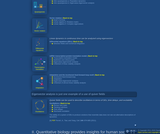Conditional Remix & Share Permitted
CC BY-SA
Rating
0.0 stars

We use eigenvector-eigenvalue analysis to walk through a simple quasispecies model described in Bull, Meyers, and Lachmann, "Quasispecies made simple," PLoS Comp Biol, 1(6):e61 (2005). The dominance of a genotype depends, not merely on its ability to breed quickly (i.e. the rudimentary concept of survival of the fittest), but also on its ability to breed "true."

Subject:
Physics
Material Type:
Lecture Notes
Provider:
Look At Physics
Provider Set:
A Mathematical Way to Think About Biology
Author:
David Liao
10/08/2012Conditional Remix & Share Permitted
CC BY-SA
Rating
0.0 stars

When a derivative of a function appears on one side of an equation and the function appears somewhere on the other side, it is common to employ a series of manipulations of notation that are called separation of variables. This strategy is often presented in a way that makes it seem as though differentials were quantities that could be independently moved around an equation. The purpose of this video is to show that the same end result can be obtained more rigorously using u-substitution, in other words, using a "change of variables."

Subject:
Physics
Material Type:
Lecture Notes
Provider:
Look At Physics
Provider Set:
A Mathematical Way to Think About Biology
Author:
David Liao
10/08/2011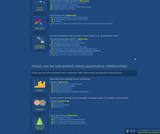Conditional Remix & Share Permitted
CC BY-SA
Rating
0.0 stars

In a toy model of a cell, protein X is produced according to a translation rate coefficient and eliminated according to a degradation rate coefficient. The protein copy number at which the rates for these processes balance is called the steady-state level, and the time it takes for a cell initially containing zero copies of protein X to accumulate half the steady-state level is called the _ŃŇrise time._Ń Surprisingly, the "rise time" depends on the degradation rate coefficient only. The classic textbook presentation of this topic is found in Alon, An Introduction to Systems Biology: Design Principles of Biological Circuits, Boca Raton: Chapman & Hall/CRC, 2007 (p. 18-22).

Subject:
Physics
Material Type:
Lecture Notes
Provider:
Look At Physics
Provider Set:
A Mathematical Way to Think About Biology
Author:
David Liao
10/08/2012Conditional Remix & Share Permitted
CC BY-SA
Rating
0.0 stars

In the first video segment, we describe the fundamental postulate of statistical mechanics. The direct product notation we introduce in the second segment helps us to discuss the states available to a collection of many parts, which helps us, in turn, to derive the Boltzmann factor in the third segment. The fourth video segment explains how the Boltzmann factor helps us to calculate average properties for systems in thermal contact with large baths and introduces entropy (Greek letter sigma), free energy (F), and the partition function (Z).

Subject:
Physics
Material Type:
Lecture Notes
Provider:
Look At Physics
Provider Set:
A Mathematical Way to Think About Biology
Author:
David Liao
10/08/2012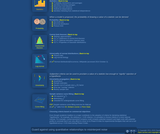Conditional Remix & Share Permitted
CC BY-SA
Rating
0.0 stars

Students will encounter the concept of a distribution, along with parameters that describe a distribution's "typical" values (average) and a distribution's spread (variance). To understand simple distributions and uncertainty propagation in the coming sections, it is necessary to be familiar with the concept of statistical independence. When two variables fluctuate independently, their covariance vanishes, and the variance of their sum is the sum of their variances.

Subject:
Physics
Material Type:
Lecture Notes
Provider:
Look At Physics
Provider Set:
A Mathematical Way to Think About Biology
Author:
David Liao
10/08/2012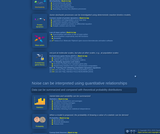Conditional Remix & Share Permitted
CC BY-SA
Rating
0.0 stars

Even when we model the dynamics of the abundances of molecules inside biological systems using calculus, it is important to remember that underlying behavior can be apparently random ("stochastic"). Even a deterministic system containing components moving in periodic ways can, at early times, support dynamics that appear disordered. The behavior of systems containing complicated collections of interacting parts can be difficult to predict with accuracy (chaos). Finally, systems can display stochasticity because the outcomes of measurements on quantum systems are indeterminate in a fundamental way. Random processes are modeled using Markov models.

Subject:
Physics
Material Type:
Lecture Notes
Provider:
Look At Physics
Provider Set:
A Mathematical Way to Think About Biology
Author:
David Liao
10/08/2012Conditional Remix & Share Permitted
CC BY-SA
Rating
0.0 stars

The purpose of the first part of this video is to introduce the idea of summation and its notation using the Greek-letter Sigma. We practice working with sums by using Gauss's summation trick. In the second part of the video, we study examples of infinite series, one which converges to a finite number (geometric series), and one which diverges (harmonic series).

Subject:
Physics
Material Type:
Lecture Notes
Provider:
Look At Physics
Provider Set:
A Mathematical Way to Think About Biology
Author:
David Liao
10/08/2012Conditional Remix & Share Permitted
CC BY-SA
Rating
0.0 stars

To continue our discussion of derivatives from preceding videos, we explain that the second derivative represents curvature. By combining knowledge of multiple derivatives, we can sometimes create Taylor series, which are local approximations of functions. As an example, we Taylor-expand sinusoidal functions and then use the results to iteratively approximate pi.

Subject:
Physics
Material Type:
Lecture Notes
Provider:
Look At Physics
Provider Set:
A Mathematical Way to Think About Biology
Author:
David Liao
10/08/2011Conditional Remix & Share Permitted
CC BY-SA
Rating
0.0 stars

Students will learn a sample-variance curve fitting method that can be used to determine whether a set of experimental data appears to have been generated by a model. This method is based on minimizing the reduced chi-squared value. This video includes a reminder to inspect normalized residuals before reporting fitted parameters.

Subject:
Physics
Material Type:
Lecture Notes
Provider:
Look At Physics
Provider Set:
A Mathematical Way to Think About Biology
Author:
David Liao
10/08/2012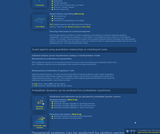Conditional Remix & Share Permitted
CC BY-SA
Rating
0.0 stars

The quadrature formula relates the fluctuations of a function to fluctuations in the variables on which the function depends. In this derivation, we approximate a multivariable function using a Taylor expansion, and we assume that fluctuations in the underlying variables are statistically independent, which allows us to apply an identity previously derived in the unit on statistics. Namely, "variances of sums are sums of variances" for variables that fluctuate independently.

Subject:
Physics
Material Type:
Lecture Notes
Provider:
Look At Physics
Provider Set:
A Mathematical Way to Think About Biology
Author:
David Liao
10/08/2012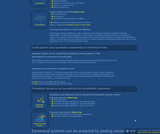Conditional Remix & Share Permitted
CC BY-SA
Rating
0.0 stars

In the first video segment, we estimate properties of a parent distribution (i.e. mean and standard deviation) from a sample of a finite collection of data measurements, and we describe the standard error (SE). In the second segment, we derive the famous square-root of n factor that appears in the SE formula. The third video segment describes the visual comparison of error bars, and the fourth segment warns against a mistake that can generate inappropriate claims of statistical significance during this kind of analysis.

Subject:
Physics
Material Type:
Lecture Notes
Provider:
Look At Physics
Provider Set:
A Mathematical Way to Think About Biology
Author:
David Liao